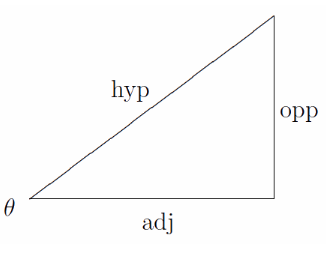# Tan Theta formula

The law of Tangent which is also called as tangent formula or tangent rule is the ratio of the sine of the angle to the cos of the angle.

Tan Θ = Opposite / Adjacent

## Tan x formula

The Tan Θ is the ratio of the Opposite side to the Adjacent, where (Θ) is one of the acute angles.

 $$\begin{array}{l}Tan \Theta = \frac{Opposite Side}{Adjacent Side}\end{array}$$## Tan alpha Questions

Out of other 6 trigonometric formulas, let’s have a look at the practice question of tan theta formula.

Example 1:

If Sin x = 4/5, Find the value of Cos x and tan x?

Solution: Using Trigonometric identities: Cos2x = 1- Sin2x

Cos2x = 1 – (4/5)2

= 1 – 16/25

= (25 – 16) / 25

= 9/25

Cos x =

$$\begin{array}{l}\sqrt{9/25}\end{array}$$

= ⅗

Tan x = Sin x / Cos x

= (4/5) / (3/5)

= 4/3

## Question on Tangent formula

Question 1: If Sec x = 4/7, find the Cos x and tan x?

Solution: As Sec x = 1/cos x

4/7 = 1/ cos x

Cos x = 7/4

Tan2 x = sec2x – 1

= (4/7)2 – 1

= 16/49 – 1

= -33 / 49

Tan x =

$$\begin{array}{l}\sqrt{-33}\end{array}$$
/7

To practice more Trigonometric questions and understand the trigonometric concepts better, Register on BYJU’S.